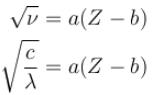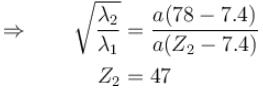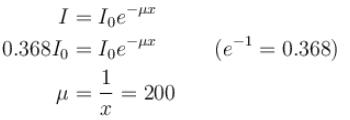Courses

# Origin Of Quantum Mechanics NAT Level – 1

## 10 Questions MCQ Test Topic wise Tests for IIT JAM Physics | Origin Of Quantum Mechanics NAT Level – 1

Description
This mock test of Origin Of Quantum Mechanics NAT Level – 1 for IIT JAM helps you for every IIT JAM entrance exam. This contains 10 Multiple Choice Questions for IIT JAM Origin Of Quantum Mechanics NAT Level – 1 (mcq) to study with solutions a complete question bank. The solved questions answers in this Origin Of Quantum Mechanics NAT Level – 1 quiz give you a good mix of easy questions and tough questions. IIT JAM students definitely take this Origin Of Quantum Mechanics NAT Level – 1 exercise for a better result in the exam. You can find other Origin Of Quantum Mechanics NAT Level – 1 extra questions, long questions & short questions for IIT JAM on EduRev as well by searching above.
*Answer can only contain numeric values
QUESTION: 1

### An X-ray tube operating at 50kV converts 1%  energy in the form of X-ray. If the amount of heat produced is 495 watts, then the number of electrons colliding with the target per second is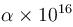. Find the value of α.

Solution:

Number of electrons colliding with the target per second is :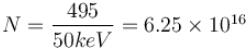*Answer can only contain numeric values
QUESTION: 2

### In a hydrogen atom following Bohr's postulates, the product of linear momentum and angular momentum is proportional to (n)x where n is the orbit number. Then x is

Solution:

Linear momentum ⇒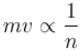Angular momentum ⇒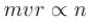∴  Product of linear momentum and angular momentum ∝ n0.

*Answer can only contain numeric values
QUESTION: 3

### Work function for aluminum metal is  4.125 eV.  For photo electric effect from aluminum metal, the cut off wavelength (in nm) will be.

Solution: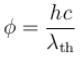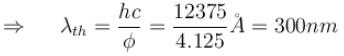*Answer can only contain numeric values
QUESTION: 4

If the short wavelength limit of the continuous spectrum coming out of a coolidge tube is 10Å, then the de-Broglie wavelength (in Å)of the electrons reaching the largest metal in the coolidge tube is approximately.

Solution: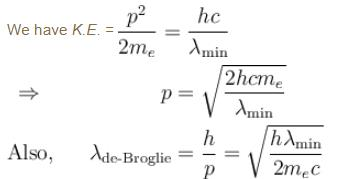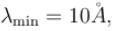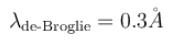*Answer can only contain numeric values
QUESTION: 5

Yellow light of 557 nm wavelength is incident on a cesium surface. It is found that no photo-electron flow in the circuit when the cathode anode voltage drops below 0.25V. Then the threshold wavelength (in nm) for photoelectric effect from cesium is.

Solution: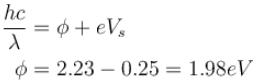⇒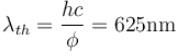*Answer can only contain numeric values
QUESTION: 6

Light of wavelength 5000Å falls on a sensitive plate with photoelectric work function of 1.9 eV. The kinetic energy (in eV) of the emitted photo-electron will be.

Solution: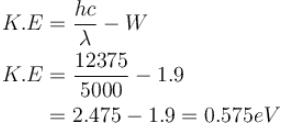*Answer can only contain numeric values
QUESTION: 7

The ratio of de-Broglie wavelength of a proton and an α-particle accelerated through the same potential difference is:

Solution: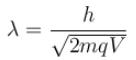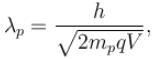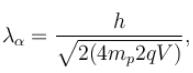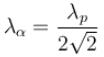⇒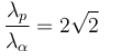*Answer can only contain numeric values
QUESTION: 8

When light of intensity 1 W/m2 and wavelength 5 × 10–7 m is incident on a surface, it is completely absorbed by the surface. If 100 photons emit one electron and area of the surface is 1 cm2, then the photoelectric current (in µA) will be.

Solution:

Number of photon/second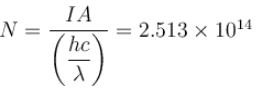Number of photon electron emitted/second by 1 photon = N/100
Photo electric current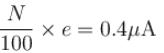*Answer can only contain numeric values
QUESTION: 9

The wavelength of Ln line in X-rays spectrum of 78Pt is 1.32 Å. The wavelength of Ln line in X-rays spectrum of another unknown element is 4.17Å. If screening constant for La line is 7.4, then atomic number of the unknown element is :

Solution: